/

### Extreme Value Theory 4. Block maxima results – examples of different limiting behaviour

The limiting behaviours of many traditional distributions are well-known. For example, block maxima values for the normal, log-normal and exponential distributions converge to the Gumbel distribution. Those for the Student’s t, inverse gamma, F and Pareto distributions converge to the Fréchet distribution.

Two examples where it is easy to derive the limiting distributions are the exponential and the Pareto distribution:

Exponential

Suppose the underlying distribution is an exponential distribution with distribution function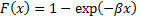for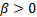and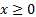. Then by choosing normalising sequences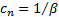and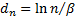we have: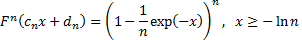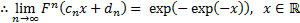So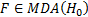.

Pareto

Suppose the underlying distribution is a Pareto distribution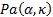with distribution function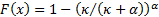for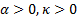and. Then by choosing the normalising sequences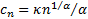and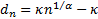we have: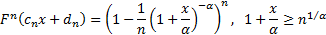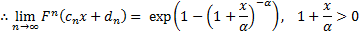So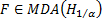.